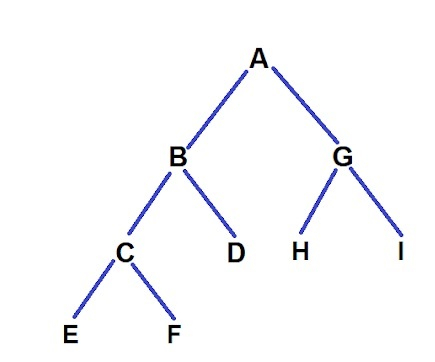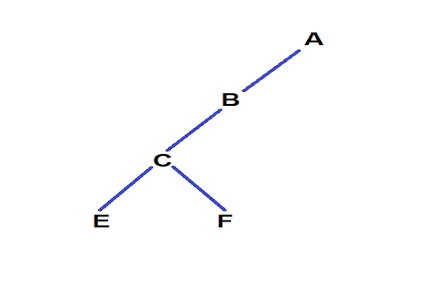# C++ Remove Nodes on Root to Leaf Paths of Length < K

C++Server Side ProgrammingProgramming

Given a tree, and we need to remove the leaf node of the path having a length less than the given k, for example.

Input −

K = 4.Output −## Explanation

The paths were :
1. A -> B -> C -> E length = 4
2. A -> B -> C -> F length = 4
3. A -> B -> D length = 3
4. A -> G -> H length = 3
5. A -> B -> I length = 3
Now as you can see paths 3, 4, 5 have length of 3 which is less than given k so we remove the leaf nodes of these paths i.e. D, H, I.
Now for path 4 and 5 when H and I are removed we notice that now G is also a leaf node with path length 2 so we again remove node G and here our program ends.

We will traverse the tree in the post-order format; then, we create a recursive function that removes our leaf nodes if their path length is less than K.

## Approach to Find the Solution

In this approach, we traverse in post-order traversal now; we try to recursively remove the leaf nodes that have path lengths less than k and continue like this.

## Example

C++ Code for the Above Approach

#include<bits/stdc++.h>
using namespace std;
struct Node{ // structure of our node
char data;
Node *left, *right;
};
Node *newNode(int data){ // inserting new node
Node *node = new Node;
node->data = data;
node->left = node->right = NULL;
return node;
}
Node *trimmer(Node *root, int len, int k){
if (!root) // if root == NULL then we return
return NULL;
root -> left = trimmer(root -> left, len + 1, k); // traversing the left phase
root -> right = trimmer(root -> right, len + 1, k); // traversing the right phase
if (!root -> left && !root -> right && len < k){
delete root;
return NULL;
}
return root;
}
Node *trim(Node *root, int k){
return trimmer(root, 1, k);
}
void printInorder(Node *root){
if (root){
printInorder(root->left);
cout << root->data << " ";
printInorder(root->right);
}
}
int main(){
int k = 4;
Node *root = newNode('A');
root->left = newNode('B');
root->right = newNode('G');
root->left->left = newNode('C');
root->left->right = newNode('D');
root->left->left->left = newNode('E');
root->left->left->right = newNode('F');
root->right->left = newNode('H');
root->right->right = newNode('I');
printInorder(root);
cout << "\n";
root = trim(root, k);
printInorder(root);
return 0;
}

## Output

E C F B D A H G I
E C F B A

## Explanation of the Above Code

In this code, we are using a recursive function that is traversing our tree and keeping the stats of the left and right subtrees. Now we arrive at a leaf node; we check the path length till that node. If the path length is less, then we delete this node, and then we return NULL; otherwise, the code continues.

## Conclusion

In this tutorial, we solve a problem to Remove nodes on the root to leaf paths of length < K using recursion. We also learned the C++ program and recursion for this problem and the complete approach we solved. We can write the same program in other languages such as C, java, python, and other languages. We hope you find this tutorial helpful.